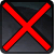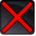# [train_aoe]Conditions

## Related effects, buffs and debuffs

• [0s] [not displayed]Effect #1

 Slot: Buff Duration: 0s Tick rate: does not tick # occurrences: 0
• On Apply

Perform the following actions:

• Unknown (183)
- Unknown (303) = (bool) true
- Unknown (306) = (bool) true
- Unknown (428) = (bool) false
- Unknown (430) = (bool) false
- Unknown (310) = (string) aoePositionOptionType_Entity
- Unknown (311) = (string) aoeTarget_Caster
- Unknown (313) = (string) aoeScalarType_LiteralValue
- Unknown (317) = (string) aoeScalarType_LiteralValue
- Unknown (321) = (string) aoeScalarType_LiteralValue
- Unknown (325) = (string) aoeOrientationOptionType_WorldCoordinates
- Unknown (344) = (string) aoeCylinder
- Unknown (349) = (string)
- Unknown (353) = (string) aoeScalarType_LiteralValue
- Unknown (354) = (string) aoeScalarType_LiteralValue
- Unknown (355) = (string) aoeScalarType_LiteralValue
- Unknown (367) = (string) aoeScalarType_LiteralValue
- Unknown (375) = (string) aoeScalarType_LiteralValue
- Unknown (384) = (string)
- Unknown (386) = (string) aoeAbilityCaster_Caster
- Unknown (387) = (string) aoeAbilityTarget_Target
- Unknown (407) = (string) aoeMovement_DirectedMotion
- Unknown (414) = (string) aoeScalarType_LiteralValue
- Unknown (418) = (string) aoeScalarType_LiteralValue
- Unknown (422) = (string) aoeScalarType_LiteralValue
- Unknown (429) = (string) aoeMovement_None
- Unknown (431) = (string) aoeRotation_None
- Ability Spec = (int) 16141122231828355160
- Unknown (307) = (int) 300
- Unknown (308) = (int) 300
- Tick Rate = (float) 0.2
- Unknown (304) = (float) 10
- Unknown (305) = (float) 10
- Unknown (314) = (float) 0
- Unknown (318) = (float) 0
- Unknown (322) = (float) 0
- Unknown (362) = (float) 0
- Unknown (363) = (float) 0
- Unknown (364) = (float) 0
- Unknown (368) = (float) 0.42
- Unknown (376) = (float) 3
- Unknown (390) = (float) 0
- Unknown (398) = (float) 0.1
- Unknown (417) = (float) 0
- Unknown (421) = (float) 0
- Unknown (425) = (float) -1
- Unknown (426) = (float) 3
- Unknown (427) = (float) 1000